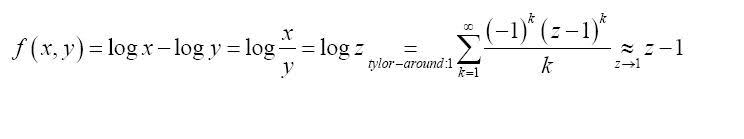# Error question

nhrock3why we do tailor around 1 and not 0
?

Last edited:

## Answers and Replies

Homework Helper
Hi nhrock3!You can "Taylor" around any value where the function is sufficiently continuous and differentiable.

But it isn't at z = 0, so you can't "Taylor" there!

(and I expect they've chosen z = 1 because it's easiest to calculate the derivatives there!)

nhrock3
so when x=y we have a problem
how transforming it into a taylor series solves this accuracy problem?

Mentor
Why do you think there is a problem when x = y? If x = y, log(x/y) = log(1) = 0.

nhrock3
the question states how to get over loss of significance problem in here
f=ln x -ln y
x,y>0
and the solution says that we have a problem when x and y are close to each other
how transforming it into a taylor series solves this accuracy problem?

Mentor
I think that the idea here is that if x is close to y, then x/y is close to 1. You could have a situation where the actual value of x/y was 1.000000000001253, but because of limitations in computing precision, you might lose the part after the zeros.

By using a Taylor series to approximate log(x) - log(y) = log(z) $\approx$ z - 1, the part after the zeros above is now significant.

nhrock3
so what if " x/y is close to 1"
"limitations in computing precision"
how does this limitation get solves by this method?

Mentor
Try it with a calculator. Enter .00000000000125, and then add 1. Many calculators will display an answer of 1 since they aren't able to maintain enough digits of precision to display the actual answer.

So if you have two numbers that are very different in relative size, (e.g. 1 vs. .00000000000125), adding them causes the loss of digits. If you can strip off the 1, though, there's no problem in storing or computing with the part to the right of all the zeros. In computers, floating point numbers are stored in a way that is similar to scientific notation. Instead of being stored as .00000000000125, it would be stored something like 1.25 X 10-12. Not exactly like that, since the base is 2, not 10, and there are some other differences.

number like 1.00000000000125, where one part is very large

nhrock3
the question states how to get over loss of significance problem in here
f=ln x -ln y?

f=ln x -ln y=ln z
the tailor series for ln z is:
$$\sum_{k=1}^{\infty}\frac{(-1)^{k+1}(Z-1)}{k}$$
but how this expression equals z-1 ?

Mentor
The summation doesn't "equal" z - 1. It's "approximately equal" to z - 1. What they have done is discard all terms of degree 2 or larger in the series.